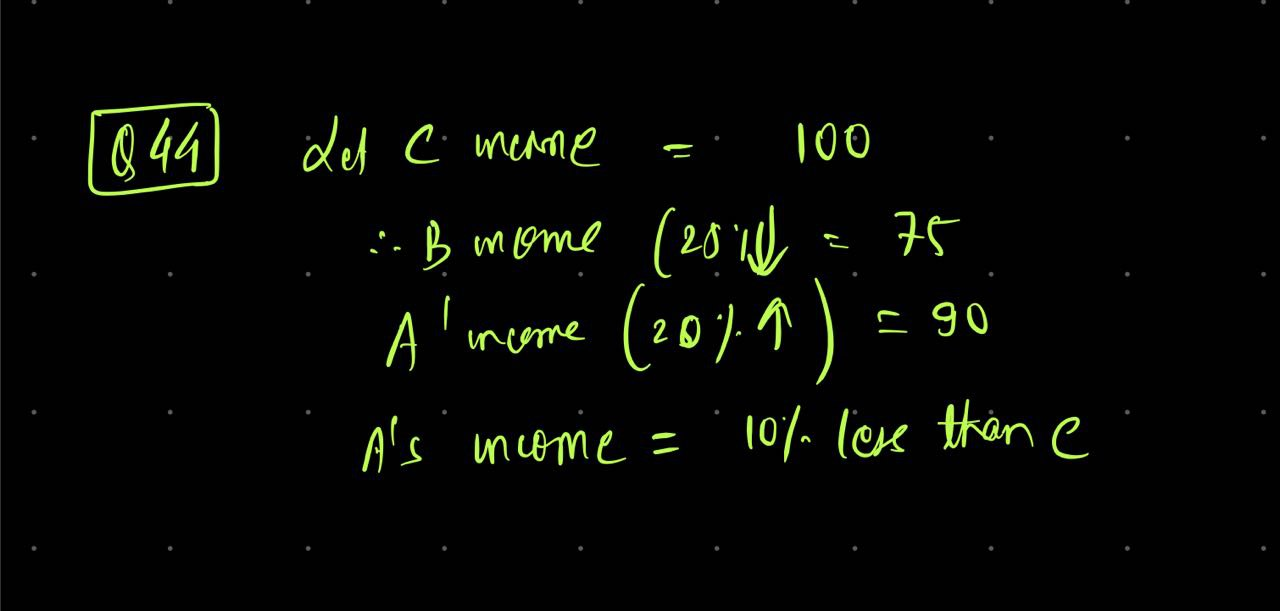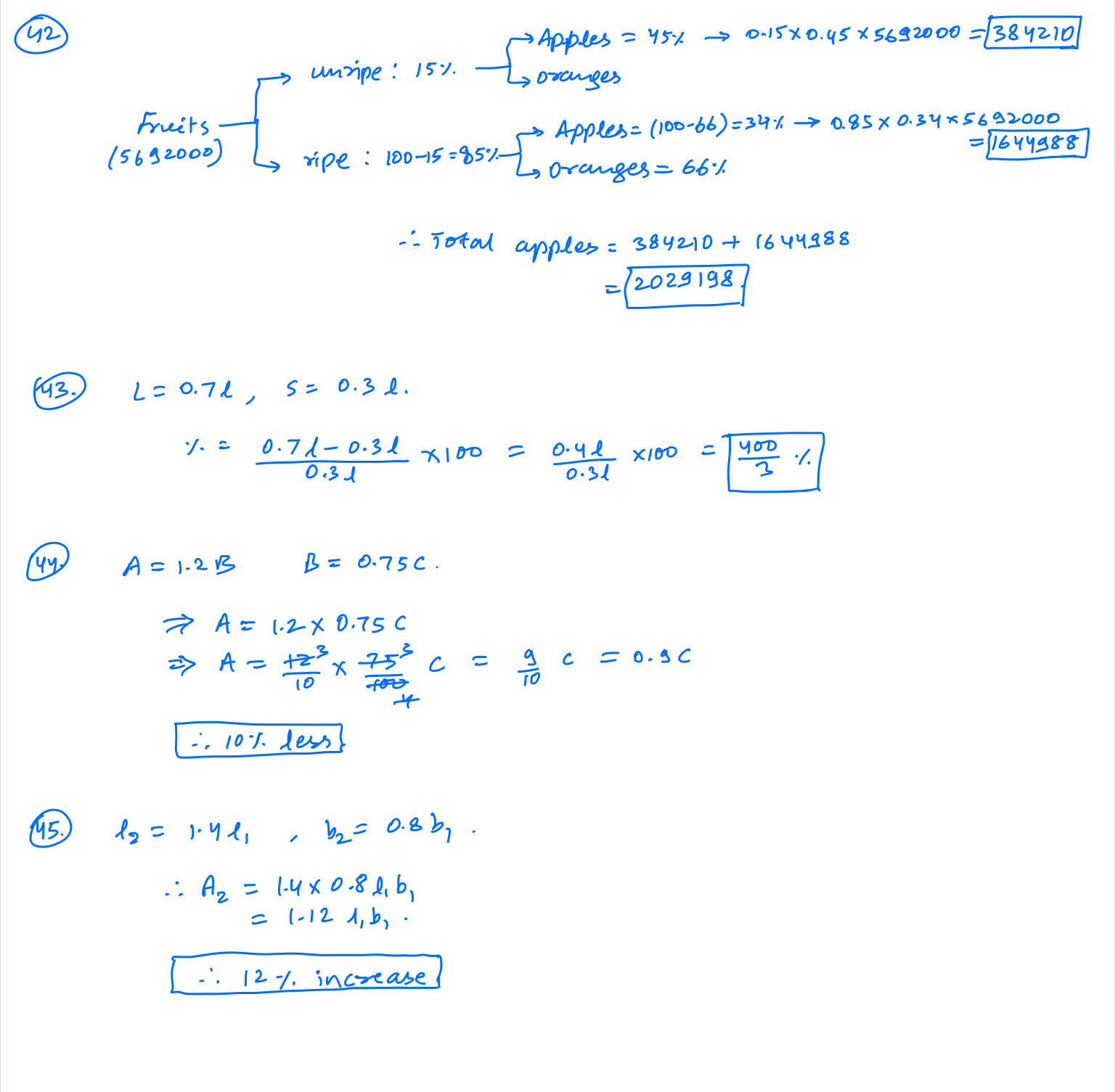CSAT 2021 Practice Questions - ForumIAS

# CSAT 2021 Practice Questions

Will be posting some csat questions for practice here on a daily basis!Neyawn,Integerand12 otherslike this
32k views

41. Price of wire is directly proportional to square of its length. Price of 10 metre is ₹1600. What is the total price of 2 wires of length 4m and 6m?

832

1.2k views

42. In a huge pile of Apple and oranges, both riped and unriped are mixed together. 15% are unriped fruits. Of the unriped, 45% are apples. Of the riped ones, 66% are oranges. If the pile contains total of 5692000 fruits, how much of them are apples?

1.2k views

43. A water pipe is cut into 2 pieces. The longer pipe is 70% of length of pipe. By how much % is the longer pipe longer than shorter piece?

1.2k views

44. Income of A is 20% higher than that of B. Income of B is 25% less than that of C. What % less is A's income than that of C?

1.2k views

45. A gardener increased area of his rectangular garden by increasing its length by 40% and decreasing its width by 20%. What is the area of new garden?

1.2k views

Alert, was reading a book and was deeply engrossed, but thought to solve it. Solution can be wrong.1.2k views
1.2k views1.2k views

@Naadan_Parinda In my defense I was testing the image uploading algorithm, to compress images whilst uploading so that page does not slow down and the new smiley from the drop down1.2k views
@Neyawn  😊 (I was also testing the smiley) never mind. Both answers are correct.

1.2k views

45. A gardener increased area of his rectangular garden by increasing its length by 40% and decreasing its width by 20%. What is the area of new garden?

Apply formula for such area change questions:

(X) + (Y) + (X) (Y) / 100

Here X = +40% Y= -20% so change in area would be

(+40) + (-20) + (+40) (-20) /100 = 40-20-8 = +12% (Area will be INCREASED by 12% !)

😊root,Naadan_Parindaand1 otherslike this
2.2k views

44. Income of A is 20% higher than that of B. Income of B is 25% less than that of C. What % less is A's income than that of C?

Change all such %age questions in p/q format , then workout with LCM method reverse engineering.

20% =1/5 , 25% = 1/4

5 and 4 ka LCM = 20 Assume this as C income

So C=20 units then B= 20×3/4=15 units

B=15 units then A= 15×6/5= 18 units

So A/C %age = (20-18)/20 ×100 = 10% !root,Naadan_Parinda
2.1k views1.2k views
@Yo_Yo_Choti_Singh These shortcuts are very less time consuming.

1.2k views

46. C.I. on sum for 2 years is ₹832 and S.I. on same sum at same rate for same period is ₹800. What is the rate of interest?

1.2k views

47. If the interest is compounded quarterly at 10% per annum. What will be the total amount at the end of 2 years if principal is ₹10,000.

1.2k views
@Yo_Yo_Choti_Singh These shortcuts are very less time consuming.

1. Practice makes u more perfect.

2. CSAT m time issues bhut kam hotey h if you know the basic techniques of attempting questions.

3. These are SSC shortcuts - converting in p/q form and LCM method makes calculation very easy and less error prone. 8×4=32 type calculations will cause less errors vis a vis 0.8×0.4 kind calculations under tensed environments.

4. But yes you need to practice well to get more perfection in these !Naadan_Parinda,
1.2k views

46. C.I. on sum for 2 years is ₹832 and S.I. on same sum at same rate for same period is ₹800. What is the rate of interest?

8%

1.2k views

47. If the interest is compounded quarterly at 10% per annum. What will be the total amount at the end of 2 years if principal is ₹10,000.

12184

1.2k viewsWrite your comment…